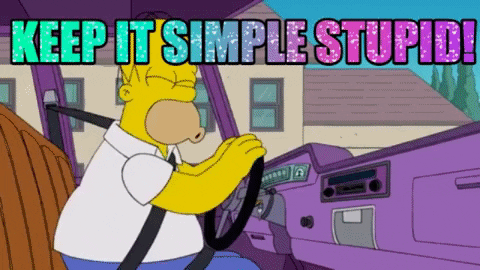# .css-df1pn7{display:block;width:16rem;}# KISS 😘 – design pattern

## Keep it simple, Stupid!

·Jul 26, 2021·

Subscribe to my newsletter and never miss my upcoming articles

Yah, that's a very emotional speech for a programming design pattern. As a side note, it tells us about the many stories in which they have struggled through complex programming code of their colleagues or even themselves from the past. keep it small and simple describes it a little better, but it sounds not so remarkable.

## Example

You may say the answer to that question is easy? But it's not what you think!

For better testing, we split our function, so that any function hast a single responsibility. So, each function is very readable and easy to test with unit tests. As an example, let's create a primitive calculator, which is able to add, subtract, multiply or divide two arguments each other.

### Good example?

``````// Add two arguments to each other
return a + b;
}

// Subtraction of two numbers
function subtract(a, b) {
return a - b;
}

// Multiply the first argument by the second
function multiply(a, b) {
return a * b;
}

// Divides the first argument by the second
function divide(a, b) {
return a / b;
}

// Delegate two numbers and their operant to the correct function
function calculator(a, b, operator) {
switch (operator) {
case '+':
case '-':
return subtract(a, b);
case '*':
return multiply(a, b);
case '/':
return divide(a, b);
default:
return 'Error';
}
}
``````

``````// caclulator which can add, subtract, multiply and divide two numbers
function calculator(a, b, operator) {
let result = 0;
switch (operator) {
case "+":
result = a + b;
break;
case "-":
result = a - b;
break;
case "*":
result = a * b;
break;
case "/":
result = a / b;
break;
}
return result;
}
``````

At first glance, the 2nd example is much easier to read, and it would be correct if there were no future with users and their new function requests, such as combining operators and rules like dot before dash. If you start to implement the new functions in that way, you will run in trouble pretty quickly.

## Summary 🌈If someone in the future ask you what the hell KISS means, do you know the answer? Probably not when it comes to the details because not everyone likes it as colourful as you do. Pair programming can help you and your friends to get better programmer with (a) KISS 💋